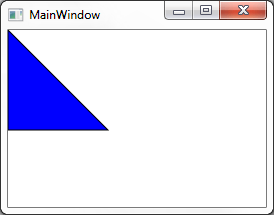Creating Shapes with PathBuilder

In a previous post I showed a quick demo of a PathBuilder class I created to help draw dynamic shapes. I would like to include a simple example of how to create Shape controls using the same tool.

I have created a very simple Triangle control which you can download and see run.

Here is the actual Triangle class

class Triangle : Shape
{
private Geometry geometry;

public static readonly DependencyProperty P1Property = DependencyProperty.Register(
"P1",
typeof(Point),
typeof(Triangle),

public static readonly DependencyProperty P2Property = DependencyProperty.Register(
"P2",
typeof(Point),
typeof(Triangle),

public static readonly DependencyProperty P3Property = DependencyProperty.Register(
"P3",
typeof(Point),
typeof(Triangle),

public Point P1
{
get { return (Point)GetValue(P1Property); }
set { SetValue(P1Property, value); }
}

public Point P2
{
get { return (Point)GetValue(P2Property); }
set { SetValue(P2Property, value); }
}

public Point P3
{
get { return (Point)GetValue(P3Property); }
set { SetValue(P3Property, value); }
}

private static void OnPointChanged(DependencyObject sender, DependencyPropertyChangedEventArgs e)
{
((Triangle)sender).UpdateGeometry();
}

private void UpdateGeometry()
{
this.geometry = PathBuilder.Start()
.Move(P1)
.DrawLine(P2)
.DrawLine(P3)
.DrawLine(P1)
.Close()
.ToGeometry();
}

public Triangle()
{
this.UpdateGeometry();
}

protected override Geometry DefiningGeometry
{
get
{
return this.geometry;
}
}
}

Notice I inherited from Shape and use the PathBuilder to generate a Geometry object and return it in the protected DefiningGeometry property.

public static Geometry ToGeometry(this IPath path)
{
var converter = new GeometryConverter();
return (Geometry)converter.ConvertFromString(path.Data);
}

This method converts the path string into a Geometry object. Here is the xaml to display this control:

<c:Triangle P1="0,0" P2="0,100" P3="100,100" StrokeThickness="1" Stroke="Black" Fill="Blue" />

And finally the Triangle in actionAuthor: justinmchase

I'm a Software Developer from Minnesota.